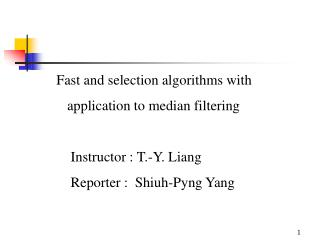DownloadDownload PresentationFast and selection algorithms with application to median filtering

# Fast and selection algorithms with application to median filtering

Télécharger la présentation## Fast and selection algorithms with application to median filtering

- - - - - - - - - - - - - - - - - - - - - - - - - - - E N D - - - - - - - - - - - - - - - - - - - - - - - - - - -
##### Presentation Transcript

1. Fast and selection algorithms with application to median filtering Instructor : T.-Y. Liang Reporter : Shiuh-Pyng Yang

2. Reference papers : • IEEE TRANSACTION ON PARALLEL AND DISTRIBUTED, VOL. 14, NO. 10, OCTOBER 2003.

3. Abstract : • The main contribution is first design fast and scalable parallel algorithms for selection and median filtering on the array with reconfigurable optical buses (AROB). • This is the most time efficient algorithm for N*N image and a W*W window, especially compared to the other median filter.

4. Outline : • Introduction • Theory • Conclusion

5. Introduction : • Median filter is a very important nonlinear filter used in image processing to eliminate noise. • In real-time image processing, such as military and industrial applications, the speed of computations is very important. • Some algorithms derived in the parallel computation models with optical buses and a straightforward serial algorithm takes

6. Computational model and basic notations : • A linear processors array with pipelined buses (LAPPB) of size N contains N processors connected the optical bus with two couplers. • One is used to write data on the upper(transmitting) segment of the bus and the other is used to read the data from the lower(receiving)segment of the bus.

7. Fig. 1. An LAPPB of size 5.

8. Fig. 2. (a) An LAROB of size N. (b) The switch states. (c) An example of bus reconfiguration.

9. Basic data manipulation operations :

10. Rotation and Inversion :

11. Fig. 3. Illustrations of rotation and inversion, N=8,W=4, u=v=1 and p=2.(a)rotation: a(1,1) is moved to a’(N,N) for x=y=1. (b) inversion: d(N+W,N+W) is moved back to d’(W+p+1,W+p+1) for x=y=1.

12. Parallel algorithms for median filtering :

13. Conclusion : • This is the first constant time median filtering algorithm to be developed on any parallel computational models. • The AROB(algorithm on the arrays with reconfigurable optical buses) is useful for solving images problems and its computational power is superior than that of other existing reconfigurable network.